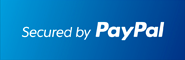# ▷Step by Step Differential Equations with the TI89 Calculator ✅### Works With TI92 Plus TI89 TI89 Titanium Voyage 200At the end of the PayPal checkout, you will be sent an email containing your key and software.Description

• The most comprehensive Differential Equations APP for calculators.
• Users have boosted their Differential Equations knowledge.
• First, Second and higher order Differential Equations.
• Shows step by step solutions for some Differential Equations such as separable, exact, ...
• Includes Slope Fields, Euler method, Runge Kutta, Wronskian, LaPlace transform, system of Differential Equations, Bernoulli DE, (non) homogeneous linear systems with constant coefficient, Exact DE, shows Integrating Factors, Separable DE and much more.
• Draw Phase Lines.
• Ideal for quick review and homework check in Differential Equation/Calculus classes.
• Easy to use. Just plug in the equation and the correct answer shows.
• Step by Step - Bernoulli Differential Equation
• Step by Step - Exact Differential Equation
• Step by Step - Non-Exact DE with Integrating Factor
• Step by Step - Homogeneous 1. Order Differential Equation
• Step by Step - Initial Value Problem Solver for 2. Order Differential Equations with non matching independent variables (Ex: y'(0)=0, y(1)=0 )
• Step by Step - Inverse LaPlace for Partial Fractions and linear numerators.
• Step by Step - LaPlace Transform (Partial Fractions, Piecewise, etc)
• Step by Step - Eigenvalue and Eigenvectors.
• Step by Step - Variation of Parameter (for 1. and 2. order Diff. Eqn's)
• Step by Step - Method of Undetermined Coefficients
• Step by Step - Wronskian to check Independence of Functions
• Step by Step - Wronskian to find Coefficients
• Step by Step - Cauchy-Euler D.E. of order 2 and 3.
• Step by Step - Riccati Equation
• Step by Step - Differential Equation Linear in x or in y (even using VOP).
• Step by Step - Linear Fractions
• Clairaut, Lagrange (d'Alembert) and Linear Fraction Differential Equations
• Solve the "Salt in the Tank" type Differential Equation problems.
• Solve any Rate problem.
• Solve RL-Circuits
• Orthogonal Trajectories
• Discrete Fourier Transform (DFT, FFT)
• Perform the Convolution of two Functions
• Compute the Infinite Binomial Series or perform the Binomial Theorem
• Vibrating Springs and solve RCL Circuits
• Find right side g(x) in y''+by'+cy=g(x) given particular solution
• Backwards Euler Method

Documentation